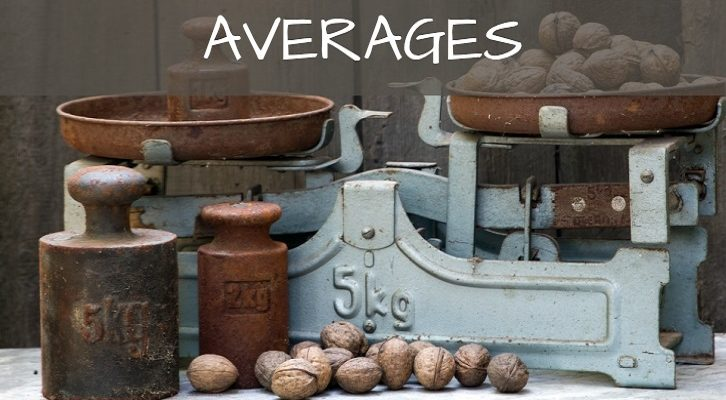# Solutions to Final few questions on mean median

Have given below solutions to the questions on mean and median .

1. The median of n distinct numbers is greater than the average, does this mean that there are more terms above the average than below it?

No.

If there are an odd number of terms, say, 2n+1, then the median is the middle term. And if average is lesser than the middle term, there will at least be n +1 terms greater than the average. So, there will be more terms above the average than below it.

However, this need not be the case when there are an even number of terms. When there are 2n distinct terms, n of them will be greater than the median and n will be lesser than the median. The average of these two terms can be such that there are n terms above the average and n below it.

For instance, if the numbers are 0, 1, 7, 7.5. The median is 4, average is 3.875. Average is less than the median. And there are more 2 numbers above the average and 2 below the average.

2. In a sequence of 25 terms, can 20 terms be below the average? Can 20 terms be between median and average?

Yes. We can have 24 zeroes and 1500 as the 25 numbers. In this case, there are 24 numbers below the average.

No. In a sequence of 25 numbers, 12 will be greater than or equal to the median and 12 will be lesser than or equal to the median. We cannot have 20 terms in between the average and median

3. From 10 numbers, a,b,c,…j, all sets of 4 numbers are chosen and their averages computed. Will the average of these averages be equal to the average of the average of the 10 numbers?

Yes. All the terms appear the same number of times when we select them 4 at a time. So, the average of averages will be equal to the overall average.